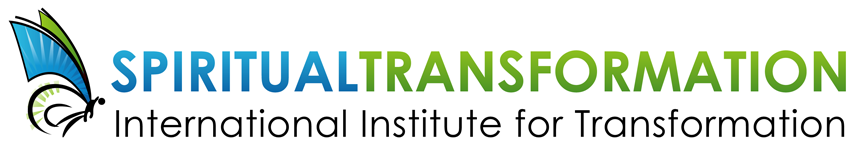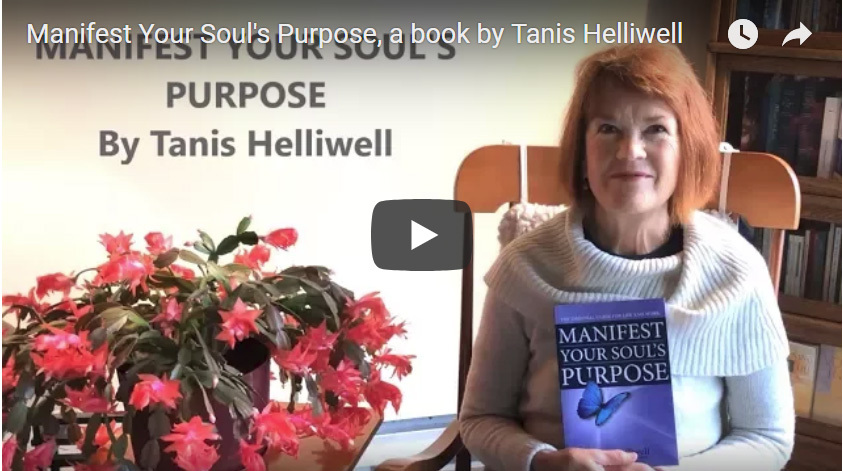# JANUARY 2018

## Happy New Year, everyone!Dear Friend,

The beginning of a new year is often a time for revisiting our life's goals. This month, a free gift and a special discount on my book, Manifest Your Soul's Purpose, to assist you with your own spiritual transformation journey.

I know it's only January, but I'm so looking forward to my workshop series scheduled throughout Europe this spring. Get a sneak peek in the link below!

 table div table+table+table+table+table div table{width:100%;padding:0}table div table+table+table+table+table div table img{width:96.23%;padding:0;float:none}table div table+table+table+table+table div table td{width:100%;padding:0 1.88% 18px}/* styles *//* styles */ “Work with the higher energy, it’s food for the angels.” ― Anonymous
 table div table+table+table+table+table+table+table div table{width:100%;padding:0}table div table+table+table+table+table+table+table div table img{width:96.23%;padding:0;float:none}table div table+table+table+table+table+table+table div table td{width:100%;padding:0 1.88% 18px}/* styles *//* styles */ Make a difference in your life, work and world, and learn to live your highest potential. In this free video, I describe how my book, Manifest Your Soul's Purpose: The essential guide for life and work will guide you in seeing how to apply spiritual truths in your everyday life -- and manifest your goals for 2018! The self-test that I refer to in the video is the Spiritual Transformation Quiz, which you can take for free here. Click on the image below to view the video or link directly here.Click on the image above to view the video. Or link directly here: https://youtu.be/yv5NTfbtkz8
 table div table+table+table+table+table+table+table+table+table+table+table div table{width:100%;padding:0}table div table+table+table+table+table+table+table+table+table+table+table div table img{width:96.23%;padding:0;float:none}table div table+table+table+table+table+table+table+table+table+table+table div table td{width:100%;padding:0 1.88% 18px}/* styles */# January special

To help manifest your soul's goals in 2018, my e-book is available at a 10% discount for the month of January.

Manifest Your Soul's Purpose is divided into three sections. The first, Balance Personality and Soul Needs, examines the different needs of the personality and soul and illustrates what "manifesting your soul's purpose" means. The second section, Transform Your Self, assists you the reader, through self-assessment exercises to determine your soul's purpose, and to examine your talents and gifts and overcome fears. The third section, Transform Your Work, offers you concrete, practical ways to bring soul into your work, and these tools will enrich your entire life.

 table.module-13{width:36.04%;padding:0}table div table+table+table+table+table+table+table+table+table+table+table+table+table+table div table{width:36.04%;float:none;margin-left:auto;margin-right:auto;padding:0}table div table+table+table+table+table+table+table+table+table+table+table+table+table+table div table a{border:0 none;text-decoration:none}table div table+table+table+table+table+table+table+table+table+table+table+table+table+table div table img{width:100%!important;border:0 none;text-decoration:none}table div table+table+table+table+table+table+table+table+table+table+table+table+table+table div table td{width:100%;padding:0}/* styles */
 /* styles */ PS: A great way to start out the new year is with our IIT Transform Yourself e-course. Find out more here, and be sure to watch the free introductory video. And learn about the signs of spiritual transformation -- find out if you are going through this transformation right now!
 table div table+table+table+table+table+table+table+table+table+table+table+table+table+table+table+table div table{width:100%;padding:0}table div table+table+table+table+table+table+table+table+table+table+table+table+table+table+table+table div table img{width:96.23%;padding:0;float:none}table div table+table+table+table+table+table+table+table+table+table+table+table+table+table+table+table div table td{width:100%;padding:0 1.88% 18px}/* styles */## 2018 European Workshops with Tanis

 table div table+table+table+table+table+table+table+table+table+table+table+table+table+table+table+table+table+table div table td,table.module-17{width:100%;padding:0}table div table+table+table+table+table+table+table+table+table+table+table+table+table+table+table+table+table+table div table{width:100%;float:none;margin-left:auto;margin-right:auto;padding:0}table div table+table+table+table+table+table+table+table+table+table+table+table+table+table+table+table+table+table div table a{border:0 none;text-decoration:none}table div table+table+table+table+table+table+table+table+table+table+table+table+table+table+table+table+table+table div table img{width:100%!important;border:0 none;text-decoration:none}/* styles */
 /* styles */ Join me this spring and summer at one of my workshops or the retreat offered in Europe. This year, we will be focusing on healing with the body elemental and working with nature spirits to heal the Earth, as well as a 5-day meditation retreat on Ancestral healing. What a joy to assist you with your spiritual transformation! For a schedule of my May/June workshops in Europe, click here. I'm so looking forward to seeing you soon!
 table div table+table+table+table+table+table+table+table+table+table+table+table+table+table+table+table+table+table+table+table div table{width:100%;padding:0}table div table+table+table+table+table+table+table+table+table+table+table+table+table+table+table+table+table+table+table+table div table img{width:96.23%;padding:0;float:none}table div table+table+table+table+table+table+table+table+table+table+table+table+table+table+table+table+table+table+table+table div table td{width:100%;padding:0 1.88% 18px}/* styles */table div table+table+table+table+table+table+table+table+table+table+table+table+table+table+table+table+table+table+table+table+table+table+table+table div table{width:100%;padding:0}table div table+table+table+table+table+table+table+table+table+table+table+table+table+table+table+table+table+table+table+table+table+table+table+table div table img{width:96.23%;padding:0;float:none}table div table+table+table+table+table+table+table+table+table+table+table+table+table+table+table+table+table+table+table+table+table+table+table+table div table td{width:100%;padding:0 1.88% 18px}/* styles */